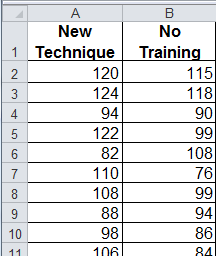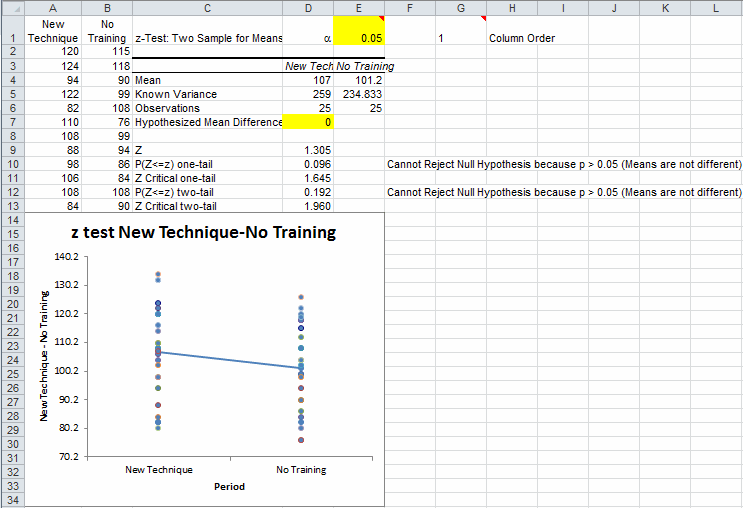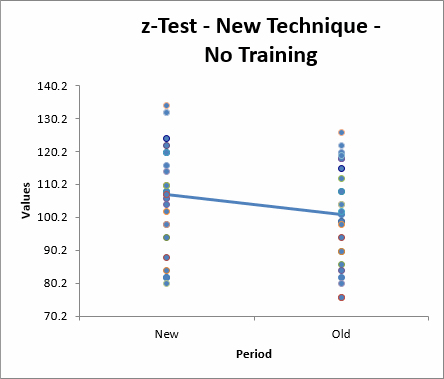# Z-Test Two Sample for Means in Excel

## QI Macros Add-in for Excel Makes Conducting Z tests a Snap

### When to use the Z-Test two sample for means

• To compare population and sample means to determine if there is a significant difference.
• To compare means between two samples.
• To compare the mean of one sample to a given constant.

The Z-test is typically used in evaluating the results of standardized tests. Are the results from a sample of students outside of or within the standard test performance?

### z Test Example: New Training Technique Designed to Increase IQ

This example was taken from Statistical Analysis in Excel for Dummies by Joseph Schmuller.

Imagine a new training technique designed to increase IQ. Take a sample of 25 people and train them using the new technique. Take another sample of 25 people and give them no special training. Input the results in Excel.#### To conduct a z test using QI Macros add-in:

1. Select the data, then click on QI Macros Menu-> Statistical Tools -> f and t tests, then Z-Test two sample for means test:2. QI Macros will prompt you to enter:
• a significance level (default = 0.05):
• a hypothesized mean difference (in this case 0 ):
3. QI Macros will calculate the variance for each data set selected and give you the opportunity to type in another value if appropriate.
4. Q Macros will perform the z test calculations AND interpret the results for you:
5.6. In the July 2022 version of QI Macros, the Box & Whisker Plot output was removed and replaced with a Values Plot output, as Values Plots provide simpler visual representations of your data.:
7.### Interpreting the z test Results

QI Macros compares the p-value (0.192) to the significance level (0.05) and interprets the result for you. In this example:"Cannot Reject the Null Hypothesis (Accept the Null Hypothesis) because p > 0.05 (Means are the Same/Means are not Different)."

• The null hypothesis H0 is that the mean difference = 0
or in other words the means are the same
• The alternative hypothesis Ha is that the mean difference is > 0
or in other words that the mean of the trained population is larger
 If Then test statistic > critical value  (i.e. z> zcrit) Reject the null hypothesis test statistic < critical value  (i.e. z< zcrit) Cannot Reject the null hypothesis (Accept the null hypothesis) p value < a Reject the null hypothesis p value > a Cannot Reject the null hypothesis (Accept the null hypothesis)

Since the null hypothesis is that the means are the same this is a two-sided test. Therefore, use the two-tail values for your analysis.

Since the z statistic < zcritical (1.305 < 1.960) and p value > a ( 0.192> 0.05) , we cannot reject the null hypothesis (accept the null hypothesis) that the means are the same.

Show less

### One-Sample Z-Test

To do a one-sample z-test on the New data above, use native Excel formulas in an empty cell:

• =ztest(A1:A26,100)
• Gives p=0.015
• Since the p-value is < 0.05, we can Reject the Null Hypothesis (Means are Different/Means are not the Same).

### Why Choose QI Macros Statistical Software for Excel?### Affordable

• Only \$329 USD - less with quantity discounts
• No annual fees
• Free Technical Support### Easy to Use

• Works Right in Excel
• Interprets Results for You
• Accurate Results Without Worry### Proven and Trusted

• 100,000 Users in 80 Countries
• Celebrating 20th Anniversary
• Five Star CNET Rating - Virus Free